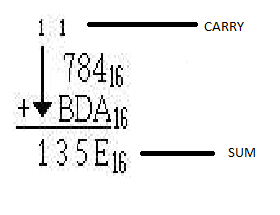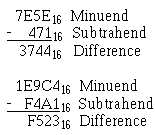## Pages

### Arithmetic For Computer : Hexadecimal Number

INTRO

• hexadecimal (hex) is a number system that has base which is 16.
• use symbol 0 - 9 to represent zero to nine and A - F to represent ten to fifteen.
• hex digit represent 4 binary digit ( bits ) or nibble.
• hex has 2 operation which are addition and subtraction.
CAUTION
never forget to put '16' after write the digit in order to distinguish between ordinary numbers and hex numbers.

• hex has 16 digit :-
0,1,2,3,4,5,6,7,8,9,A,B,C,D,E,F = 0,1,2,3,4,5,6,7,8,9,10,11,12,13,14,15
• if the sum is 16, carry '1' will move to the next digitexample of addition for hexadecimal number

SUBTRACTION

• it follow the following rules :-
1. convert to binary numbers
2. find 2nd complement
4. convert to hex
5. drop carry (if have)
• if the value is smaller, 'borrow' from the next digit (same as primary school's subtract operation)some examples for subtraction for hexadecimal number

below is a video showing us how to make both hex addition and subtraction operation

that's all from me
wait for another entry
hope that this will help........
p/s = practice make perfect!